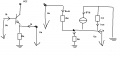# Small signal analysis of common collector circuit

#### uhf737

Joined Dec 9, 2017
14
Hello.
I am having a bit of a Problem solving this task. I need to calculate Rin, Rout and the current gain Ai ("e" is in and "a" is out)This is how I tried to solve it :

1)

Re = ReT = Ue/ie for Ua=0

Ue = ie*rbe+Re*(ie+ie*ß) solved for Ue/ie : Re = rbe + (1+ß)Re

2)

Ra = Ua/ia for Ue = 0

Ua = Re*(ube/rbe+ube/rbe*ß - Ua/rce+ia) for ube = -Ua/rbe

Ua = Re*(-Ua/rbe - Ua/rbe*ß - Ua/rce +ia) solved for Ua/ia = Re/(1+(1+ß)*Re/rbe+Re/rce)

3)

Ai = ia/ie for Ua=0

ie = ib
ia = Ua/Re - ib - ib*ß

ia/ie = -ib - ib*ß/ib = - ib*ß

I think 3) is obviously wrong since the current shouldnt be inverted...

Last edited:

#### WBahn

Joined Mar 31, 2012
26,398
Where are you taking the bias conditions into account?

#### uhf737

Joined Dec 9, 2017
14
I'm kind of lost here, what do you mean by bias conditions ? be has to be positive voltage while cb has to be negative voltage ?

#### uhf737

Joined Dec 9, 2017
14
The input/output resistance seems to be correct judging by the solution I have but the current gain is definitely off.

EDIT: Maybe there is something wrong with the way I am going at it. 1. Step : disconnect all DC sources, Step 2 : rewrite the circuit in a way that simplifies further calculations

Next is the Input Impedance which is the input voltage divided by the current ie that I feed into the transistor (Vout is zero for this calculcation)

Output Impedance is the output voltage divided the current I feed into the exit of the transistor (Vin is zero for this calculation)

Voltage gain is simply Vout/Vin which is about 1 for this circuit

Current gain is output current/input current (output voltage is zero for this calculation)

Last edited:

#### Jony130

Joined Feb 17, 2009
5,252
Look at this exampel

Zin = Vin/Iin

So, from the KVL ( II Kirchhoff's law ) we will have:

Vin = Ib*rbe + Ie*Re||rce